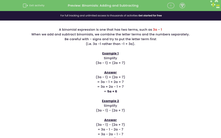# Practise Adding and Subtracting Two Algebraic Terms

In this worksheet, students will add and subtract binomial expressions.Key stage:  KS 3

Curriculum topic:   Algebra

Curriculum subtopic:   Simplify Algebraic Expressions to Maintain Equivalence

Popular topics:   Algebra worksheets

Difficulty level:#### Worksheet Overview

A binomial expression is one that has two terms, such as 3a - 1

When we add and subtract binomials, we combine the letter terms and the numbers separately.

Be careful with negative signs and try to put the letter term first, for instance,  3a -1 rather than -1 + 3a.

Example 1

Simplify:

(3a - 1) + (2a + 7)

(3a - 1) + (2a + 7)

= 3a - 1 + 2a + 7

= 3a + 2a - 1 + 7

= 5a + 6

Example 2

Simplify:

(3a - 1) - (2a + 7)

(3a - 1) - (2a + 7)

= 3a - 1 - 2a - 7

= 3a - 2a - 1 - 7

= a - 8

Example 3

Simplify:

(3a - 1) - (2a - 7)

(3a - 1) - (2a - 7)

= 3a - 1 - 2a +

Did you spot that we had two negative signs which makes a positive?

= 3a - 2a - 1 + 7

= a + 6

Let's have a go at some questions now.

### What is EdPlace?

We're your National Curriculum aligned online education content provider helping each child succeed in English, maths and science from year 1 to GCSE. With an EdPlace account you’ll be able to track and measure progress, helping each child achieve their best. We build confidence and attainment by personalising each child’s learning at a level that suits them.

Get started